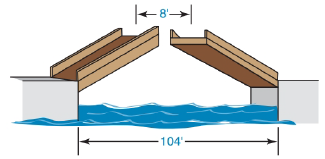Chapter 5.4, Problem 20E### Elementary Geometry for College St...

6th Edition
Daniel C. Alexander + 1 other
ISBN: 9781285195698

#### Solutions

Chapter
Section### Elementary Geometry for College St...

6th Edition
Daniel C. Alexander + 1 other
ISBN: 9781285195698
Textbook Problem
6 views

# A drawbridge that is 136 ft in length is raised at its midpoint so that the uppermost points are 16 ft apart. How far has each of the midsections been raised?(Hint: Consider the drawing for Exercise 19.)To determine

To Find:

Length of the midsections been raised.

Explanation

Given A drawbridge that is 136 ft. in length is raised at its midpoint so that the uppermost points are 16 ft. apart.

The figure can be redrawn as,

From the given data,

AB=136 ft.

CD=EF=16 ft.

And from the redrawn figure,

AE = FB

AC=12(AB)    =12(136)    =68

And from the figure,

AB=AE+EF+FB

### Still sussing out bartleby?

Check out a sample textbook solution.

See a sample solution

#### The Solution to Your Study Problems

Bartleby provides explanations to thousands of textbook problems written by our experts, many with advanced degrees!

Get Started

#### In problems 63-73, factor each expression completely. 71.

Mathematical Applications for the Management, Life, and Social Sciences

#### 23-42 Find f. f(x)=4x3

Calculus (MindTap Course List)

#### find the real roots of each equation by factoring. 129. x2 + x 12 = 0

Applied Calculus for the Managerial, Life, and Social Sciences: A Brief Approach

#### Differentiate the function. y=x3(2+x)

Single Variable Calculus: Early Transcendentals

#### A normal vector to the plane 2x + 3y + 6z =–20 is:

Study Guide for Stewart's Multivariable Calculus, 8th

#### The polar coordinates of the point P in the figure at the right are:

Study Guide for Stewart's Single Variable Calculus: Early Transcendentals, 8th

#### Define the concept of external validity and a threat to external validity.

Research Methods for the Behavioral Sciences (MindTap Course List)